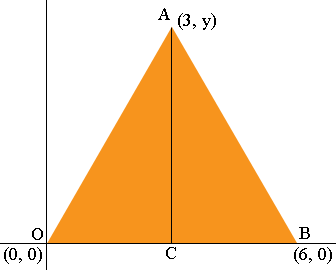SEARCH HOMEMath Central Quandaries & QueriesQuestion from Meagan, a student: An equilateral triangle has vertices at (0,0) and (6,0) in a coordinate plane. What are the coordinates of the third vertex? You may want to sketch it out. Note: The sides of an equilateral triangle are identical in length.H Meagan,

I drew your triangle. The line segment AC bisects the angle BAO.Triangles BAC and CAO are congruent and hence |OC| = |CB| and angle ACB is a right angle. Hence the x-coordinate of A is 3.Hence the right triangle CBA has one sid of length 3 and a hypotenuse of length 6. Use Pythagoras theorem to find the length of the third side, which is the y-coordinate of A.

HarleyMath Central is supported by the University of Regina and The Pacific Institute for the Mathematical Sciences.